Surface Area of Cuboid

Chapter 11 Class 8 Mensuration
Concept wise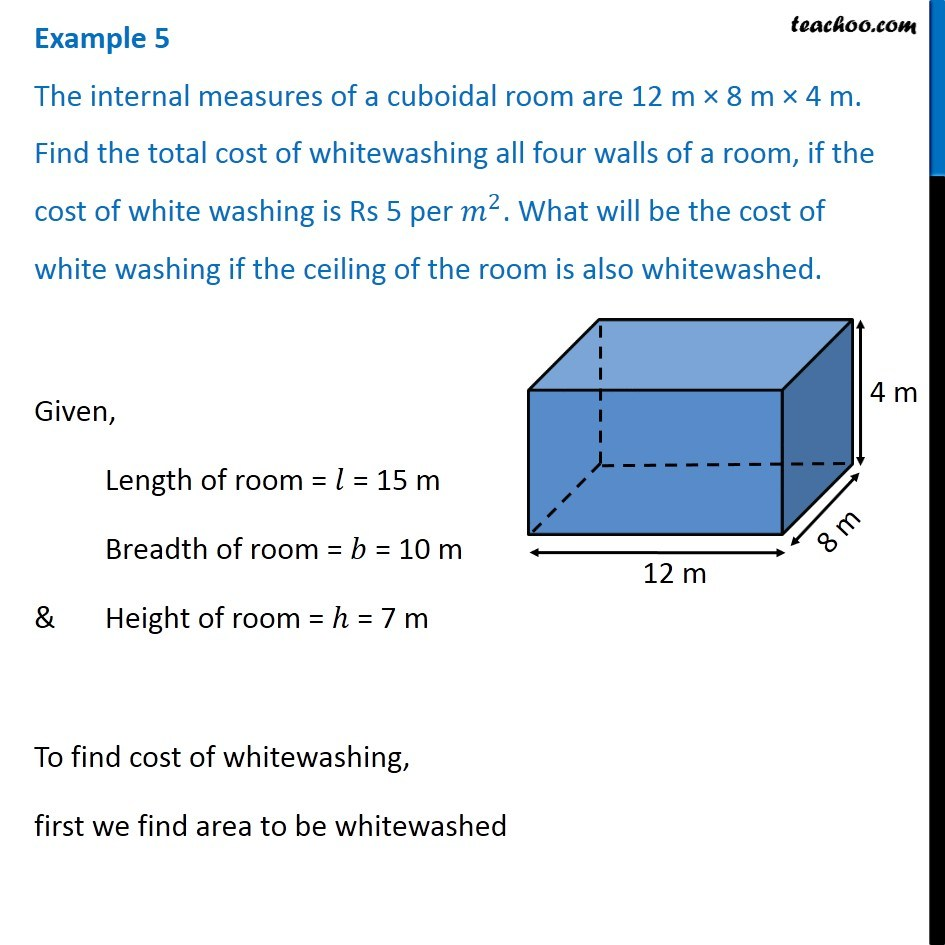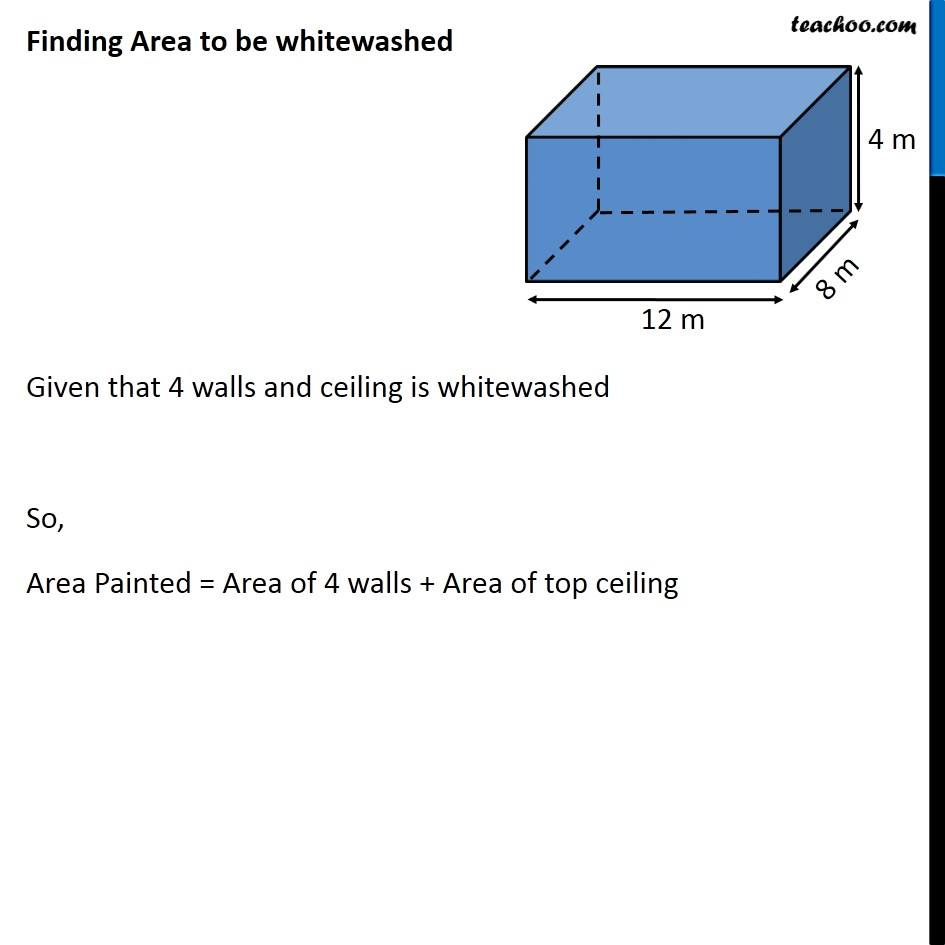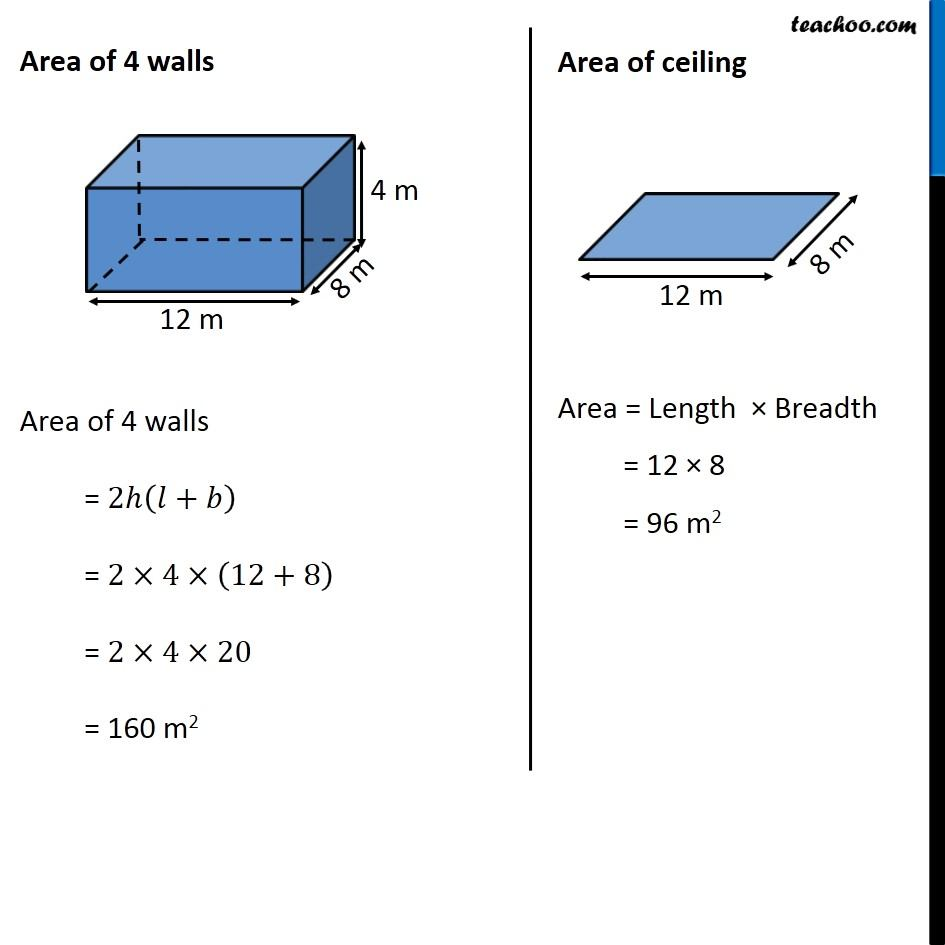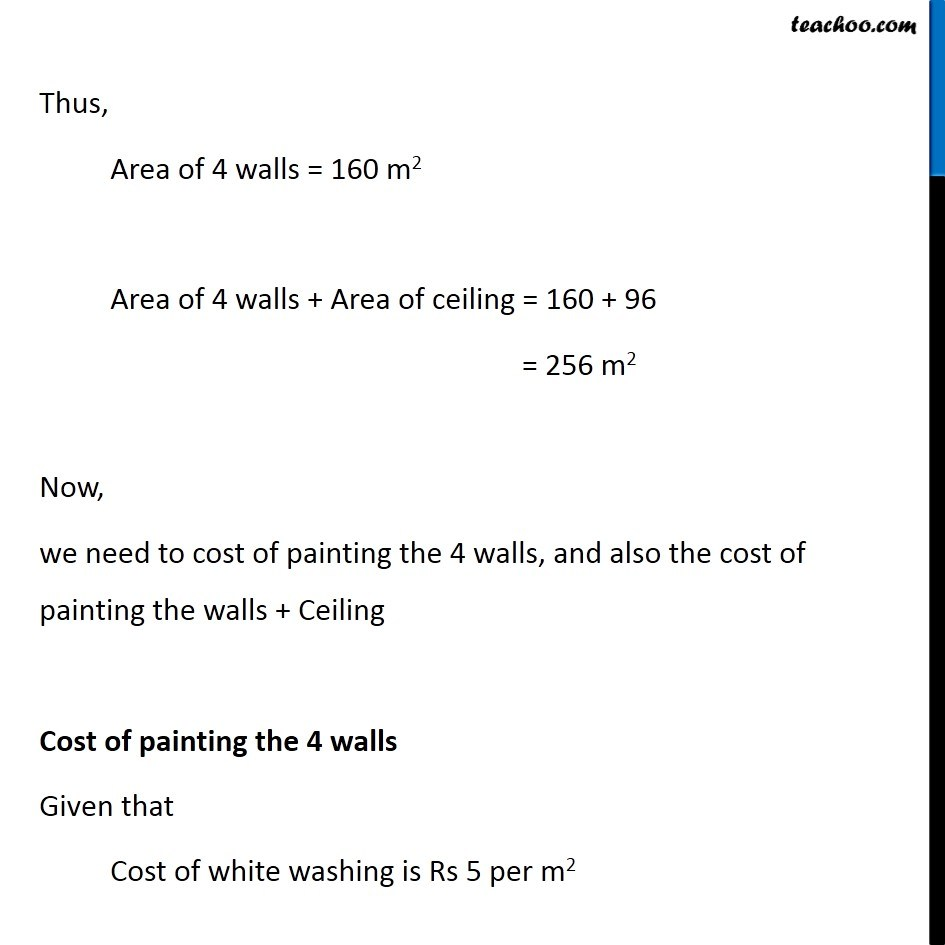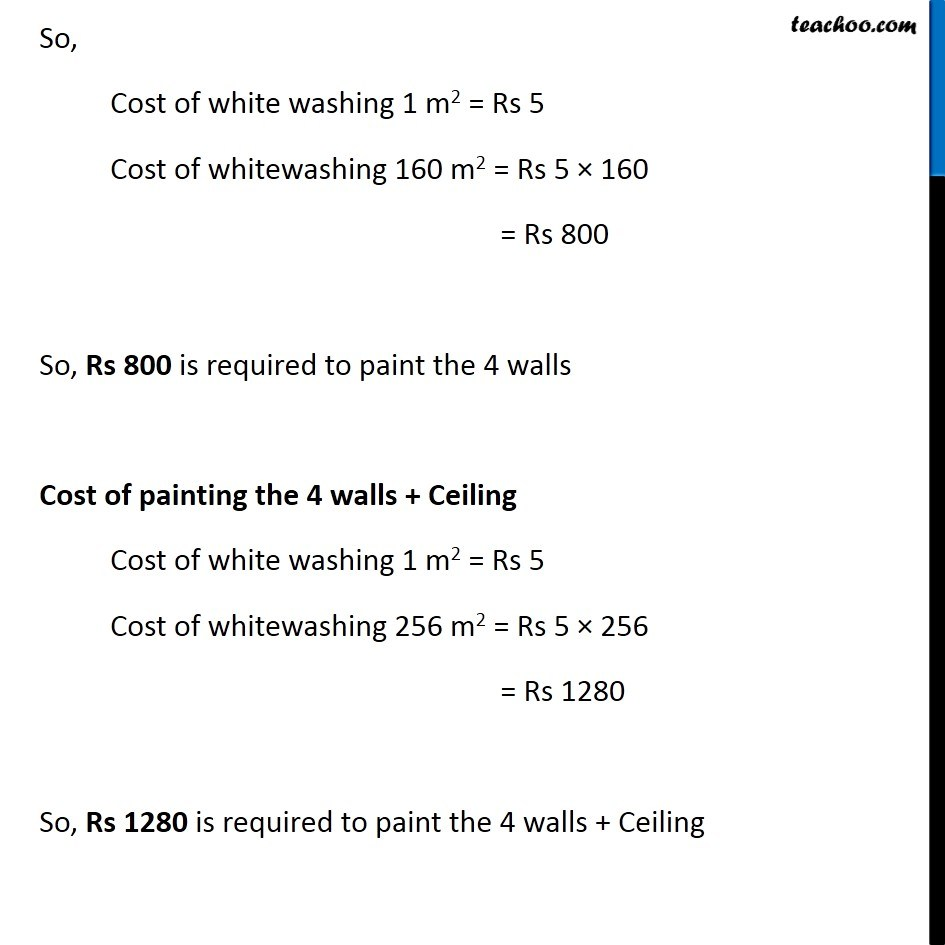Introducing your new favourite teacher - Teachoo Black, at only ₹83 per month

### Transcript

Example 5 The internal measures of a cuboidal room are 12 m × 8 m × 4 m. Find the total cost of whitewashing all four walls of a room, if the cost of white washing is Rs 5 per 𝑚^2. What will be the cost of white washing if the ceiling of the room is also whitewashed. Given, Length of room = 𝑙 = 15 m Breadth of room = 𝑏 = 10 m & Height of room = ℎ = 7 m To find cost of whitewashing, first we find area to be whitewashed Finding Area to be whitewashed Given that 4 walls and ceiling is whitewashed So, Area Painted = Area of 4 walls + Area of top ceiling Area of 4 walls Area of 4 walls = 2ℎ(𝑙+𝑏) = 2×4×(12+8) = 2×4×20 = 160 m2 Area of ceiling Area = Length × Breadth = 12 × 8 = 96 m2 Thus, Area of 4 walls = 160 m2 Area of 4 walls + Area of ceiling = 160 + 96 = 256 m2 Now, we need to cost of painting the 4 walls, and also the cost of painting the walls + Ceiling Cost of painting the 4 walls Given that Cost of white washing is Rs 5 per m2 So, Cost of white washing 1 m2 = Rs 5 Cost of whitewashing 160 m2 = Rs 5 × 160 = Rs 800 So, Rs 800 is required to paint the 4 walls Cost of painting the 4 walls + Ceiling Cost of white washing 1 m2 = Rs 5 Cost of whitewashing 256 m2 = Rs 5 × 256 = Rs 1280 So, Rs 1280 is required to paint the 4 walls + Ceiling